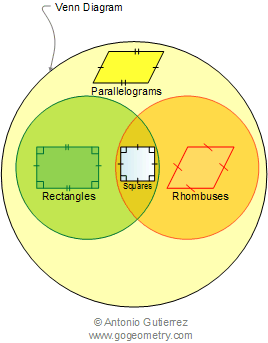Parallelogram Definition

 PARALLELOGRAM: is a quadrilateral whose opposite sides are parallel. trapezoid is a quadrilateral having two parallel sides called bases.SPECIAL PARALLELOGRAMS: RECTANGLE, RHOMBUS, SQUARE Rectangle: is an equiangular parallelogram. Rhombus: is an equilateral parallelogram. Square: is an equilateral and equiangular parallelogram. Venn Diagram of the special parallelogramsHome | Search | Geometry | Parallelogram | Definitions | Area of a Parallelogram | Email | by Antonio Gutierrez